# Selina Solutions Concise Maths Class 10 Chapter 6 Solving (simple) Problems (Based On Quadratic Equations) Exercise 6(E)

Combinations of previous exercises, concepts are put into this exercise to test the students. This ensures a student can face any problem involving quadratic equations. The Selina Solutions for Class 10 Maths prepared by expert faculty team at BYJU’S has all the solutions to problems to help students strengthen their problem-solving skills. Solutions to these other miscellaneous problems in this exercise are available in the Concise Selina Solutions for Class 10 Maths Chapter 6 Solving (simple) Problems (Based On Quadratic Equations) Exercise 6(E) PDF given below.

## Selina Solutions Concise Maths Class 10 Chapter 6 Solving (simple) Problems (Based On Quadratic Equations) Exercise 6(E) Download PDF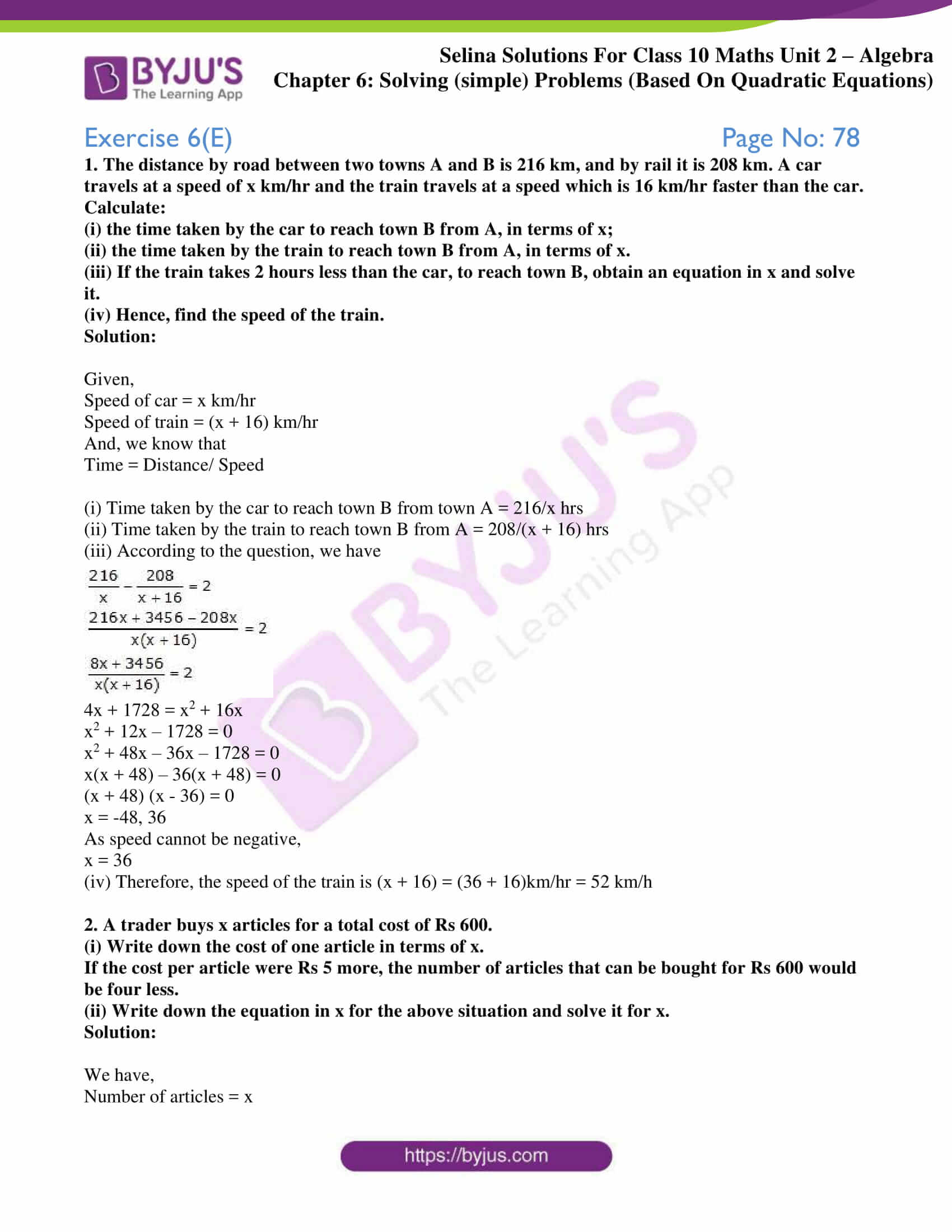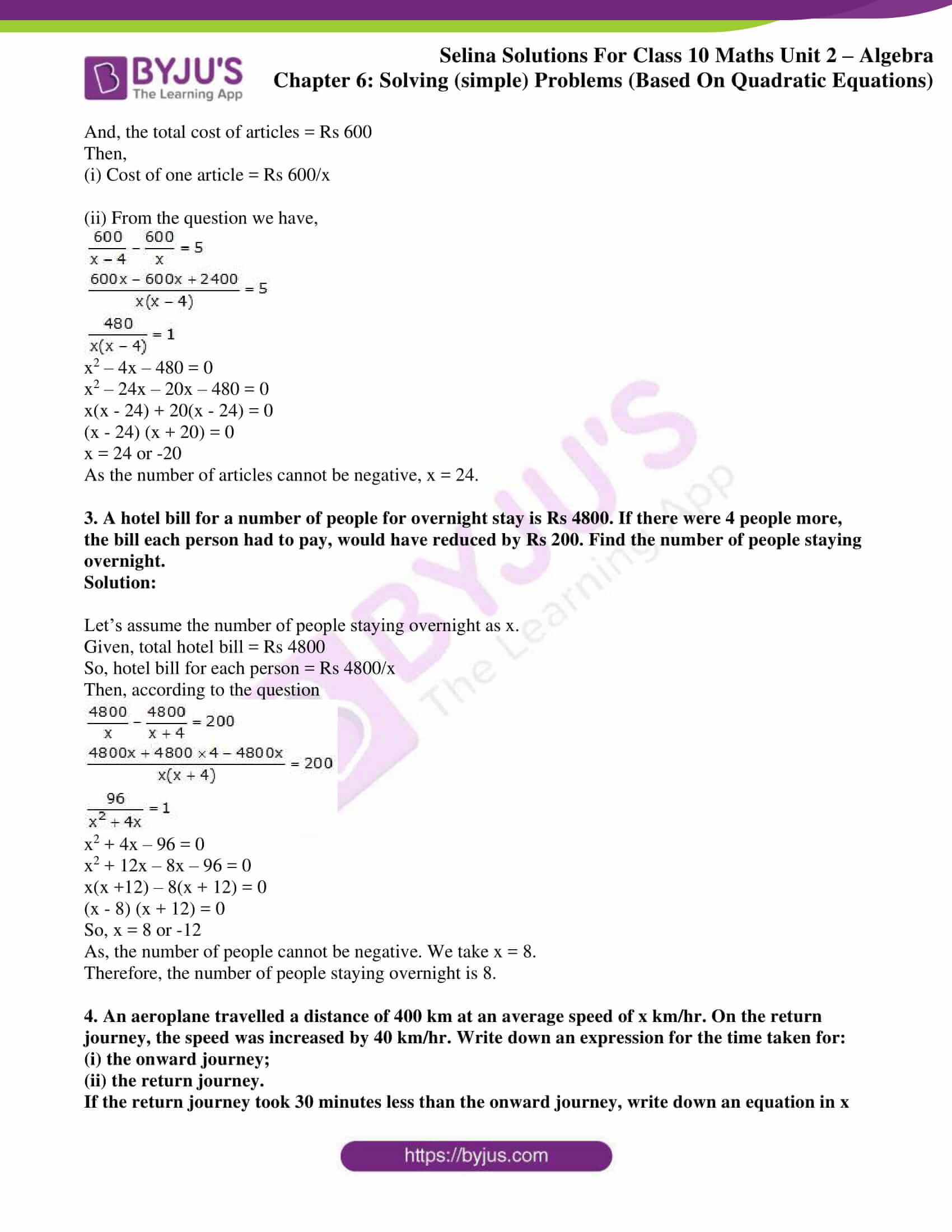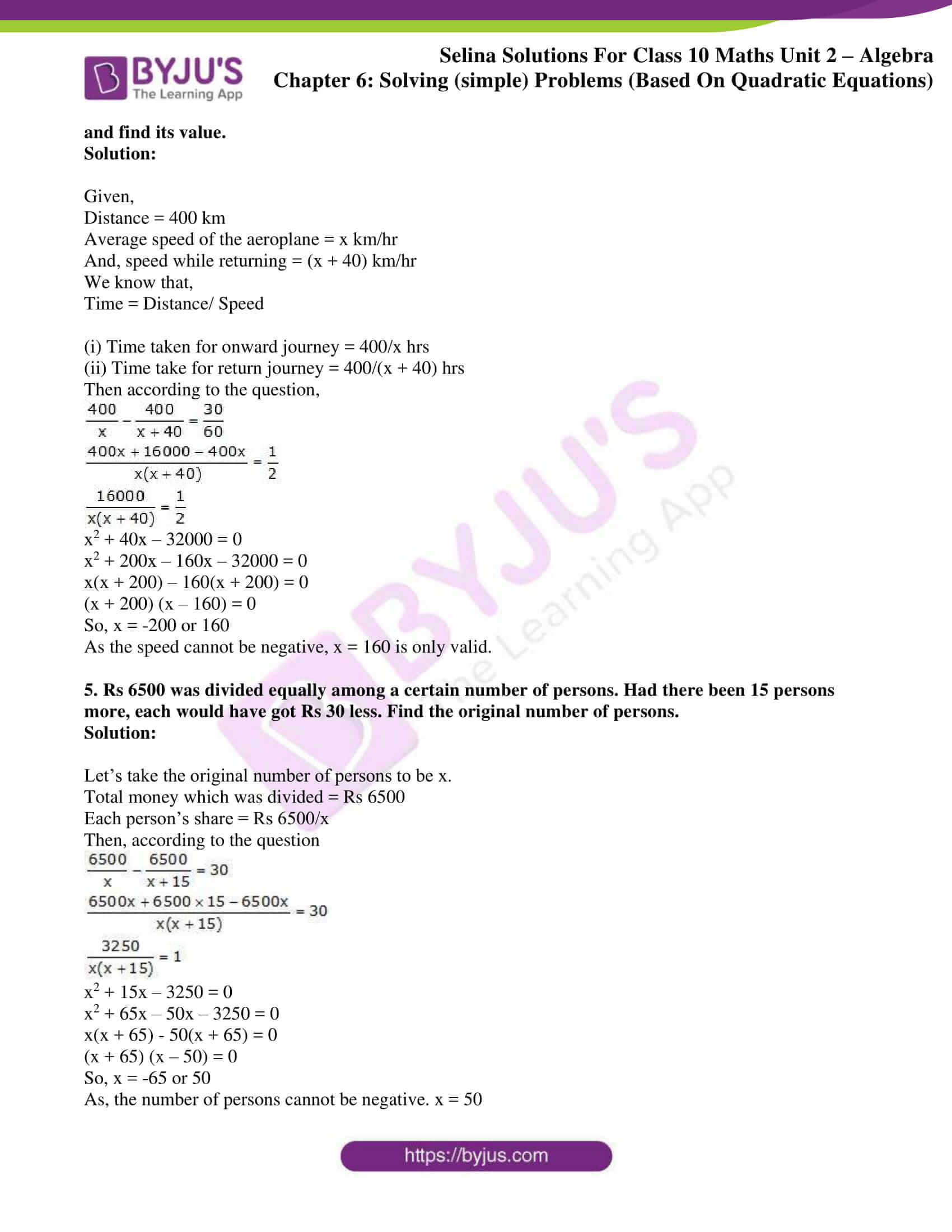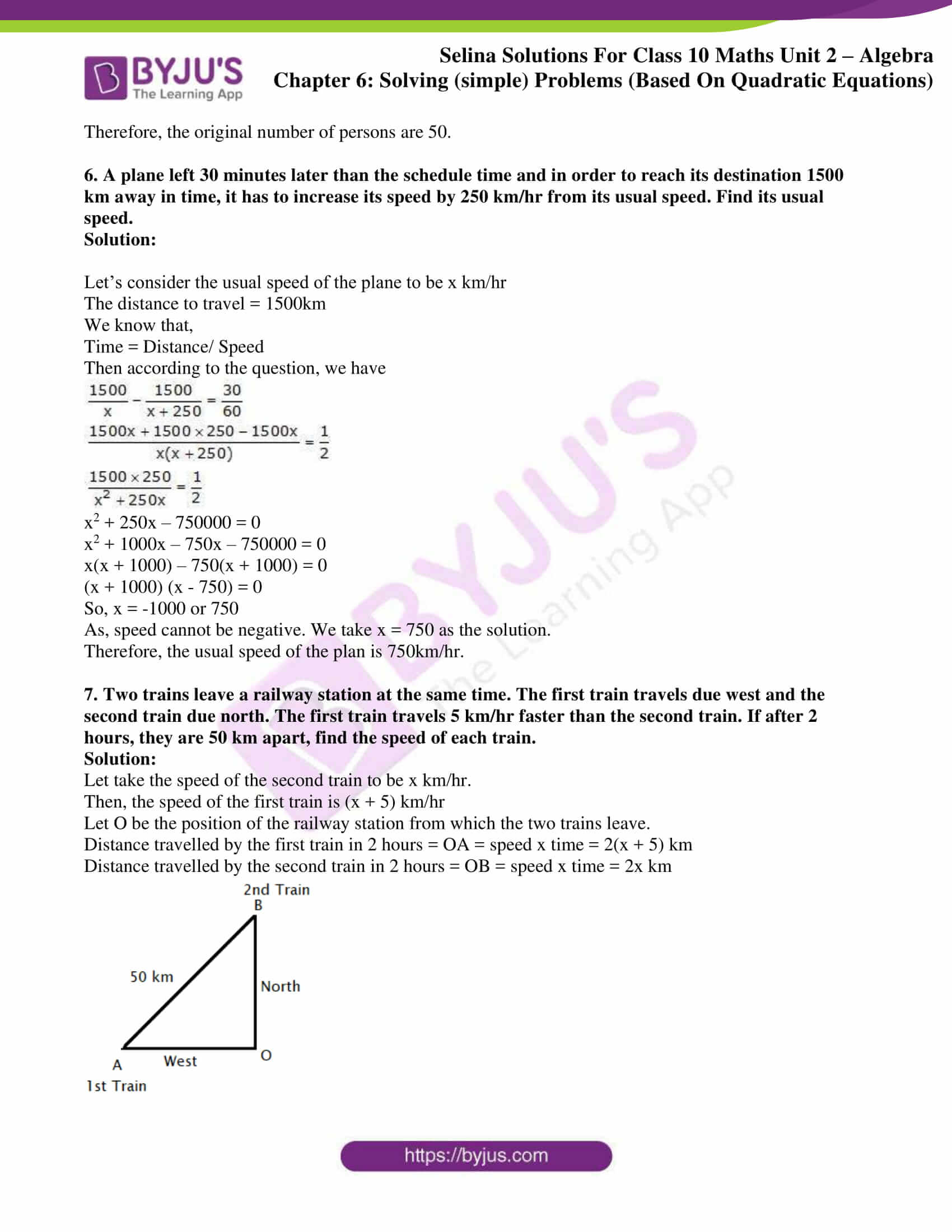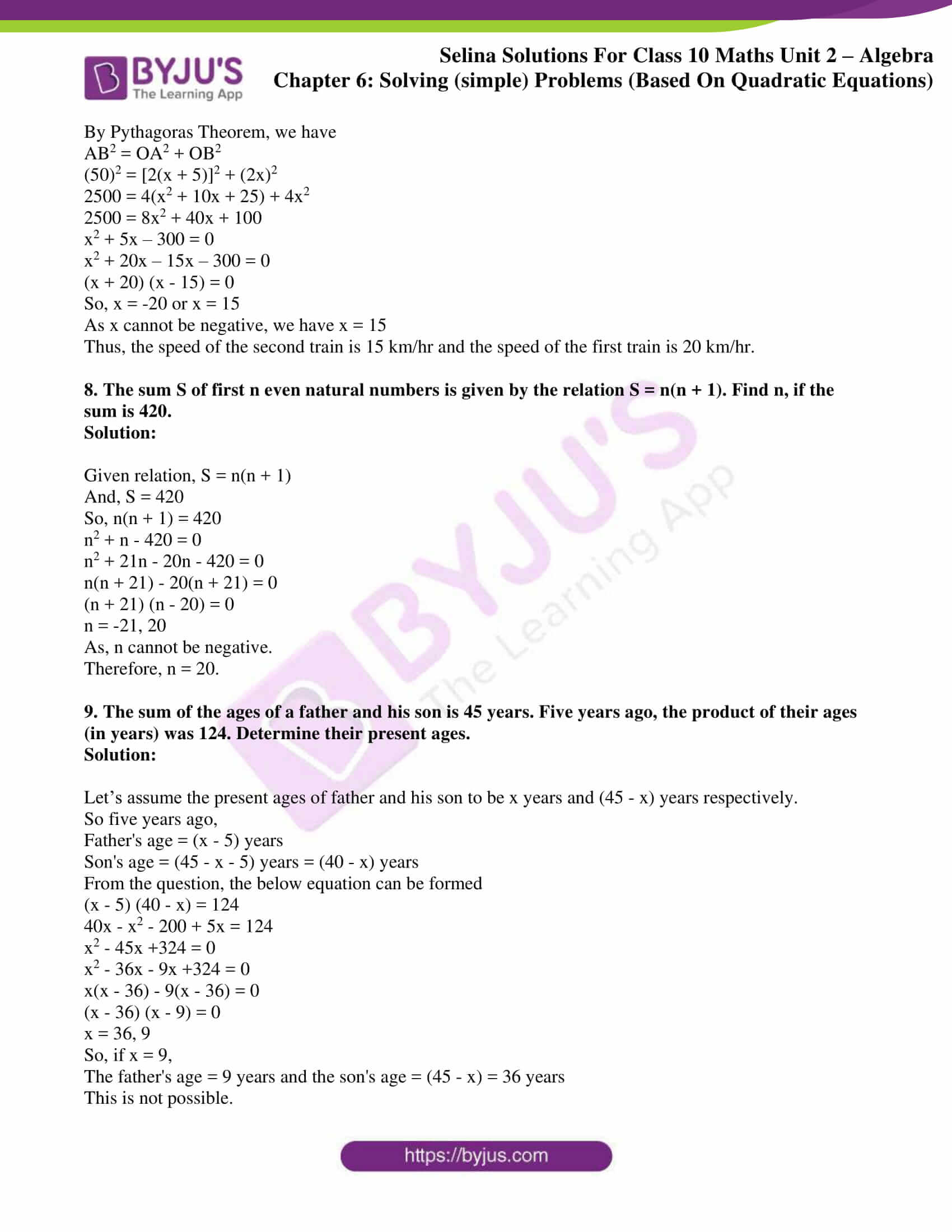### Access Selina Solutions Concise Maths Class 10 Chapter 6 Solving (simple) Problems (Based On Quadratic Equations) Exercise 6(E)

1. The distance by road between two towns A and B is 216 km, and by rail it is 208 km. A car travels at a speed of x km/hr and the train travels at a speed which is 16 km/hr faster than the car. Calculate:

(i) the time taken by the car to reach town B from A, in terms of x;

(ii) the time taken by the train to reach town B from A, in terms of x.

(iii) If the train takes 2 hours less than the car, to reach town B, obtain an equation in x and solve it.

(iv) Hence, find the speed of the train.

Solution:

Given,

Speed of car = x km/hr

Speed of train = (x + 16) km/hr

And, we know that

Time = Distance/ Speed

(i) Time taken by the car to reach town B from town A = 216/x hrs

(ii) Time taken by the train to reach town B from A = 208/(x + 16) hrs

(iii) According to the question, we have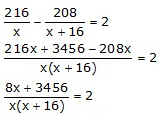4x + 1728 = x2 + 16x

x2 + 12x – 1728 = 0

x2 + 48x – 36x – 1728 = 0

x(x + 48) – 36(x + 48) = 0

(x + 48) (x – 36) = 0

x = -48, 36

As speed cannot be negative,

x = 36

(iv) Therefore, the speed of the train is (x + 16) = (36 + 16)km/hr = 52 km/h

2. A trader buys x articles for a total cost of Rs 600.

(i) Write down the cost of one article in terms of x.

If the cost per article were Rs 5 more, the number of articles that can be bought for Rs 600 would be four less.

(ii) Write down the equation in x for the above situation and solve it for x.

Solution:

We have,

Number of articles = x

And, the total cost of articles = Rs 600

Then,

(i) Cost of one article = Rs 600/x

(ii) From the question we have,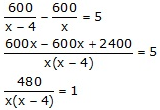x2 – 4x – 480 = 0

x2 – 24x – 20x – 480 = 0

x(x – 24) + 20(x – 24) = 0

(x – 24) (x + 20) = 0

x = 24 or -20

As the number of articles cannot be negative, x = 24.

3. A hotel bill for a number of people for overnight stay is Rs 4800. If there were 4 people more, the bill each person had to pay, would have reduced by Rs 200. Find the number of people staying overnight.

Solution:

Let’s assume the number of people staying overnight as x.

Given, total hotel bill = Rs 4800

So, hotel bill for each person = Rs 4800/x

Then, according to the question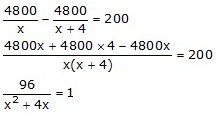x2 + 4x – 96 = 0

x2 + 12x – 8x – 96 = 0

x(x +12) – 8(x + 12) = 0

(x – 8) (x + 12) = 0

So, x = 8 or -12

As, the number of people cannot be negative. We take x = 8.

Therefore, the number of people staying overnight is 8.

4. An aeroplane travelled a distance of 400 km at an average speed of x km/hr. On the return journey, the speed was increased by 40 km/hr. Write down an expression for the time taken for:

(i) the onward journey;

(ii) the return journey.

If the return journey took 30 minutes less than the onward journey, write down an equation in x and find its value.

Solution:

Given,

Distance = 400 km

Average speed of the aeroplane = x km/hr

And, speed while returning = (x + 40) km/hr

We know that,

Time = Distance/ Speed

(i) Time taken for onward journey = 400/x hrs

(ii) Time take for return journey = 400/(x + 40) hrs

Then according to the question,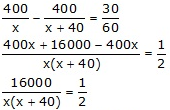x2 + 40x – 32000 = 0

x2 + 200x – 160x – 32000 = 0

x(x + 200) – 160(x + 200) = 0

(x + 200) (x – 160) = 0

So, x = -200 or 160

As the speed cannot be negative, x = 160 is only valid.

5. Rs 6500 was divided equally among a certain number of persons. Had there been 15 persons more, each would have got Rs 30 less. Find the original number of persons.

Solution:

Let’s take the original number of persons to be x.

Total money which was divided = Rs 6500

Each person’s share = Rs 6500/x

Then, according to the question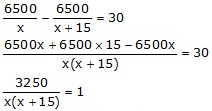x2 + 15x – 3250 = 0

x2 + 65x – 50x – 3250 = 0

x(x + 65) – 50(x + 65) = 0

(x + 65) (x – 50) = 0

So, x = -65 or 50

As, the number of persons cannot be negative. x = 50

Therefore, the original number of persons are 50.

6. A plane left 30 minutes later than the schedule time and in order to reach its destination 1500 km away in time, it has to increase its speed by 250 km/hr from its usual speed. Find its usual speed.

Solution:

Let’s consider the usual speed of the plane to be x km/hr

The distance to travel = 1500km

We know that,

Time = Distance/ Speed

Then according to the question, we have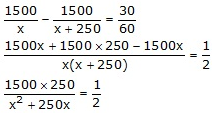x2 + 250x – 750000 = 0

x2 + 1000x – 750x – 750000 = 0

x(x + 1000) – 750(x + 1000) = 0

(x + 1000) (x – 750) = 0

So, x = -1000 or 750

As, speed cannot be negative. We take x = 750 as the solution.

Therefore, the usual speed of the plan is 750km/hr.

7. Two trains leave a railway station at the same time. The first train travels due west and the second train due north. The first train travels 5 km/hr faster than the second train. If after 2 hours, they are 50 km apart, find the speed of each train.

Solution:

Let take the speed of the second train to be x km/hr.

Then, the speed of the first train is (x + 5) km/hr

Let O be the position of the railway station from which the two trains leave.

Distance travelled by the first train in 2 hours = OA = speed x time = 2(x + 5) km

Distance travelled by the second train in 2 hours = OB = speed x time = 2x km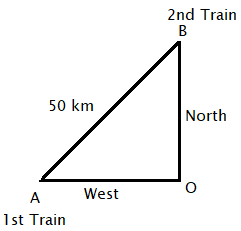By Pythagoras Theorem, we have

AB2 = OA2 + OB2

(50)2 = [2(x + 5)]2 + (2x)2

2500 = 4(x2 + 10x + 25) + 4x2

2500 = 8x2 + 40x + 100

x2 + 5x – 300 = 0

x2 + 20x – 15x – 300 = 0

(x + 20) (x – 15) = 0

So, x = -20 or x = 15

As x cannot be negative, we have x = 15

Thus, the speed of the second train is 15 km/hr and the speed of the first train is 20 km/hr.

8. The sum S of first n even natural numbers is given by the relation S = n(n + 1). Find n, if the sum is 420.

Solution:

Given relation, S = n(n + 1)

And, S = 420

So, n(n + 1) = 420

n2 + n – 420 = 0

n2 + 21n – 20n – 420 = 0

n(n + 21) – 20(n + 21) = 0

(n + 21) (n – 20) = 0

n = -21, 20

As, n cannot be negative.

Therefore, n = 20.

9. The sum of the ages of a father and his son is 45 years. Five years ago, the product of their ages (in years) was 124. Determine their present ages.

Solution:

Let’s assume the present ages of father and his son to be x years and (45 – x) years respectively.

So five years ago,

Father’s age = (x – 5) years

Son’s age = (45 – x – 5) years = (40 – x) years

From the question, the below equation can be formed

(x – 5) (40 – x) = 124

40x – x2 – 200 + 5x = 124

x2 – 45x +324 = 0

x2 – 36x – 9x +324 = 0

x(x – 36) – 9(x – 36) = 0

(x – 36) (x – 9) = 0

x = 36, 9

So, if x = 9,

The father’s age = 9 years and the son’s age = (45 – x) = 36 years

This is not possible.

Hence, x = 36

Therefore,

The father’s age = 36 years

The son’s age = (45 – 36) years = 9 years